Cuboctahedron
 What is a Cuboctahedron? Special Views Variables of the Cuboctahedron Properties Net Rainbow Cube Cuboctahedron on the Internet References
 To the Main Page    "Mathematische Basteleien"

What is a Cuboctahedron?
A cuboctahedron is a figure, which is formed by six squares and eight equilateral triangles.

Two views follow: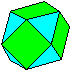A square and a triangle are lying parallel to the drawing plane.
Therefore these figures are true to the original.

Obviously the name cuboctahedron is formed by the words cube and octahedron.
 ...... If you set squared pyramids on the faces of the cube, you can imagine that a (green) cube and a (red) octahedron penetrate each other.  The intersection is the cuboctahedron.

The cuboctahedron belongs to the 13 Archimedean solids. I made a page (in German only).

Those who know how to use the 3D-View, can see the cuboctahedron threedimensionally.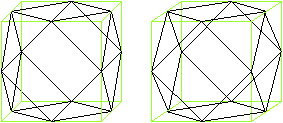You can recognize that the cuboctahedron can develop from a (green) cube. You connect the centres of the cube edges. Thus eight pyramids arise, which you must cut off. The remaining solid is the cuboctahedron.

Special Views    topVariables of the Cuboctahedron  top
The length of the edge of the cuboctahedron is given as a.
Then you can calculate the Radius R of the circumscribed sphere, the volume V, the area O, the distance  d3 of the triangles, and the distance d4 of the squares.
There isDerivations
Area
If a is the length of the edge of the cuboctahedron, the surface ist O=6*a²+8*(1/4)sqrt(3)a²=[6+2sqrt(3)]a².
You use the area formulas A=(1/4)sqrt(3)a² of the equilateral triangle and A=a² of the square.

Volume
The producing cube has the edge length sqrt(2)a.
Subtract the volumes of the eight triangle pyramids from the volume of the producing cube.

Volume of the pyramidThe formula is V=base*height/3. The triangle in front is chosen as the base.  There is V'=(a²/4)*[(1/2)sqrt(2)a]/3=(1/24)sqrt(2)a³.
Thus the volume of the cuboctahedron is V=2sqrt(2)a³-8V' = 2sqrt(2)a³-8(1/24)sqrt(2)a³ = (5/3)sqrt(2)a³.

Thickness
Two squares lie in opposite to each other.
Their distance is d1=sqrt(2)a.

Two triangles lie in opposite to each other.
Their distance d2 is the length of the space diagonal of the cube sqrt(3)[sqrt(2)a]=sqrt(6)a, decreased by the double height of the corner pyramid. The height h is related to the base "eqilateral triangle". There is V'=[(1/4)sqrt(3)a²]h/3=(1/12)sqrt(3)a²h or V'=(1/24)sqrt(2)a³ (see above) for the volume of the triangle pyramid. Thus there is h=(1/6)sqrt(6)a.
The searched distance is d2=sqrt(6)a-2(1/6)sqrt(6)a = (2/3)sqrt(6)a.

Circumscribed sphere R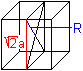There is only drawn the producing cube. You see R=a.
If you know the hexagons inside the cuboctahedron,  R=a is simpler to be seen (below).

Inscribed sphere
There is no inscribed sphere. It has to touch all squares and triangles. But the distances are different.

Properties    top
Building an octahedron.
You can put together all eight triangle pyramids, which were cut off the producing cube. They fit the cube.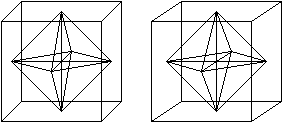Hexagons
There are pairs of triangles at the cuboctahedron. In the middle planes there are regular hexagons as borders.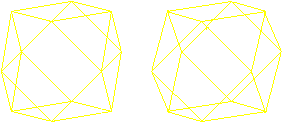There are four different hexagons.
Now you see, why a circumscribed sphere exists. Its centre is the centre of the cuboctahedron, the radius is the edge length.

Closest packing of spheres
If you lay around one (red) sphere six equal spheres and lay in the dips three more spheres above and below, then the centres of the (grey) spheres are the corners of a cuboctahedron.This leads to the closest packing of spheres in space. 12 spheres touch a central sphere.
In 2D six circles touch a central circle. 24 hyperspheres touch a central hyperspheres in the fourdimensional case.
Numbers like 6,12,24 are called "kissing numbers".

Euler's path
Four edges meet at the corners of the cuboctahedron. Therefore it is possible to follow the edges, so that you use the edges once only.
You find more on my page House of Santa Claus.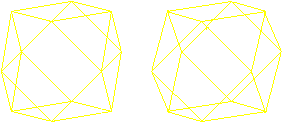Net       top
If you spread out the cuboctahedron, you get the following net from six squares and eight triangles. This is one example.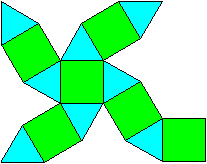You see: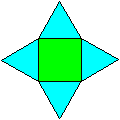Every square is surrounded by four equilateral triangles.Every triangle is surrounded by three squares.

There is a template in the book (1) "M.C.Escher Kaleidozyklen".Rainbow Cube   top
 ...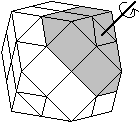... The cuboctahedron has become a puzzle similar to Rubik's Cube. It is from Japan.  There are middle planes between the two triangles and the hexagon. Thus you get a slice to every triangle, which you can turn around an axis, which goes through the centre of the cubeoctahedron and is perpendicular to the triangle.  On the left there is one of the eight slices drawn in grey.

 ...... The surface of the cuboctahedron is coloured in a similar way to Rubik's Cube. Opposite areas have the same colours.  If you turn some of the eight slices, the puzzle is out of order. There is the aim now to reproduct one colour for all squares and triangles.
This is the puzzle: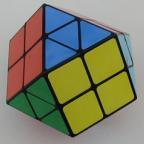Cuboctahedron on the Internet    top

German

Claus Michael Ringel
Kuboktaeder

Geneviève Tulloue ( Figures Animées pour la Physique )
Polyhedra (Applets)

H. B. Meyer (Polyeder aus Flechtstreifen)
Kubo-Oktaeder

English

Eric W. Weisstein     (MathWorld)
Cuboctahedron, Triangular CupolaArchimedean Solid, Kissing NumberOctahemioctahedron
Cubohemioctahedron

George W. Hart
Virtual Polyhedra (The Encyclopedia of Polyhedra)

Gijs Korthals Altes
Paper model Cuboctahedron

H. B. Meyer (Polyhedra plaited with paper strips)
Cuboctahedron

Jaap Scherphuis
Rainbow Cube

Kenneth James Michael MacLean
THE CUBEOCTAHEDRON

Poly
Die meisten Zeichnungen auf dieser Seite entstanden mit Hilfe dieses Programms.

French

Robert FERRÉOL
CUBOCTAÈDRE

References       top
(1) Doris Schattenschneider und Wallace Walker, M.C.Escher Kaleidozyklen, Köln 1992

Feedback: Email address on my main page